# Piezo Bender Energy Harvester

This example shows how to model a device that harvests energy from a vibrating object by using a piezo bender. The device uses this energy to charge a battery and power a load.

These devices are common in low-power applications that require energy autonomy, such as wearable devices or sensors in vehicles.

In this example, the vibration sources from which the energy is harvested are: * *Sinusoidal* - The vibration source is an engine of a vehicle rotating at constant speed. * *Chirp signal* with increasing or decreasing frequency - The vibration source is an engine that is ramping up or down in speed.

### Model Overview

This energy harvester consists of a piezo bender, a rectifier, and a DC-DC converter.

The left end of the piezo bender is clamped to a vibrating object, forcing the motion. The right end of the piezo bender is connected to an extra mass. Due to the elasticity, mass, and inertia of the piezo bender, the motion of the right end is not synchronous to the left end. The deformations produce then a charge and voltage across the electrical terminals of the piezo bender, that are harvested into power.

The full-wave rectifier transforms the AC power generated by the piezo bender into DC power. It comprises four diodes and a capacitor that acts as a filter to smooth the DC voltage.

The buck converter regulates the voltage to transfer the maximum possible power to the load and ensures that the transfer of power is unidirectional. In this example a pulse generator controls the converter in open-loop with a fixed switching frequency and duty cycle. If the vibration source does not have a constant frequency or it contains harmonics, you can design a more sophisticated closed-loop controller to optimize the transfer of power and improve the efficiency of the energy harvester in different conditions.

Initially, the energy harvester charges a battery. Then both the energy harvester and the battery power up a constant power load.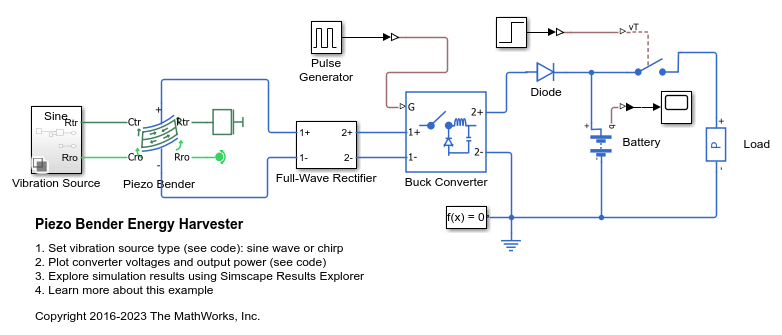### Plot Results for Sinusoidal Source

The plot below shows the results of a vibration source oscillating at a constant frequency

```Output power at final time = 68.7664 mW ```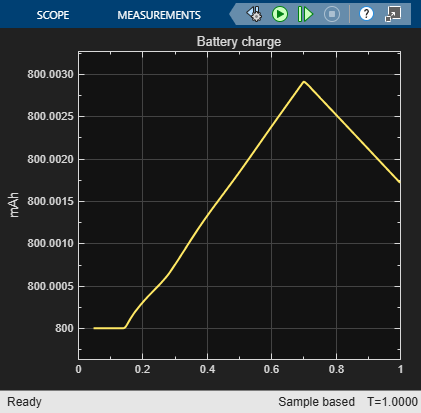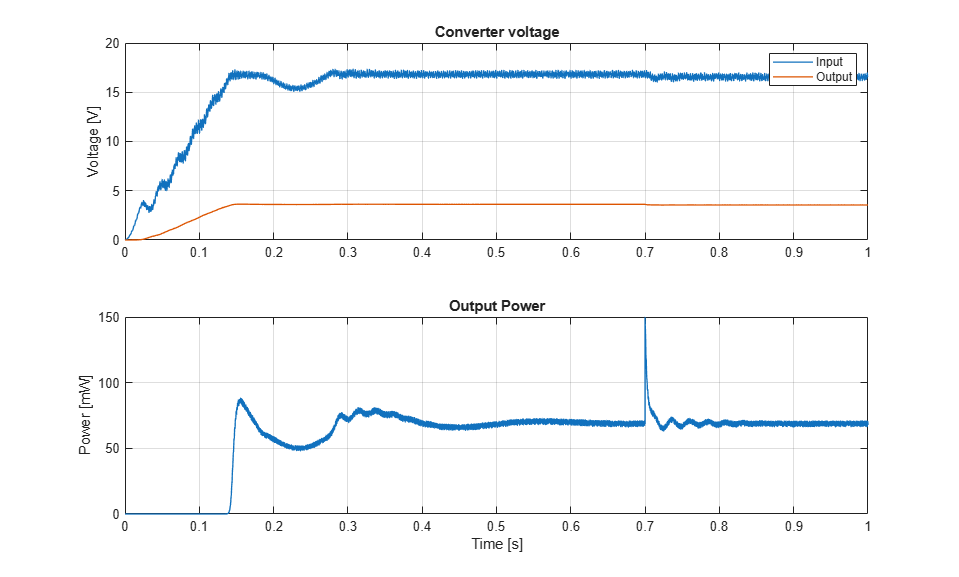From a mechanical perspective, piezoelectric benders are flexible beams with a natural fundamental frequency of oscillation. When a piezo bender oscillates at this resonant frequency, it generates the maximum power.

The frequency of the source is close to the resonant frequency, so it's generating almost the maximum possible power for this energy harvester.

### Plot Results for Chirp Source

The plot below shows the results of a vibration source with a linearly-increasing frequency

```Output power at final time = 0.71323 mW ```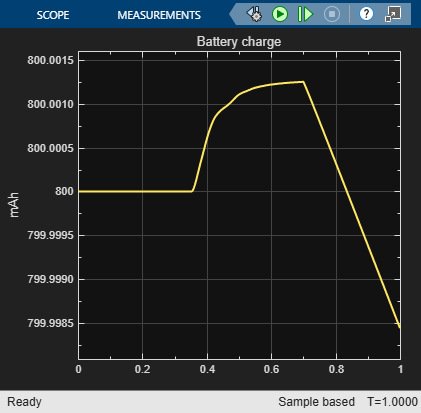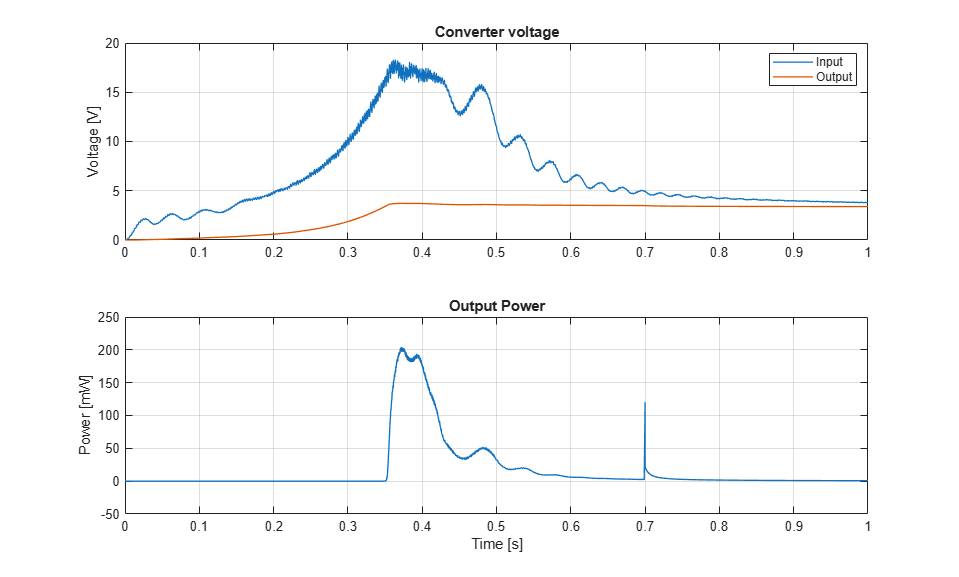The output power increases as the source is approaching the resonant frequency. Then, it decreases as the source exceeds the resonant frequency.